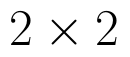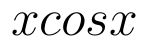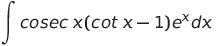Select Page

# MCQ Answers for CBSE 12 Science Maths Matrices in English

MCQ Answers for CBSE 12 Science Maths Matrices in English to enable students to get Answers in a narrative video format for the specific question.

Expert Teacher provides MCQ Answers for CBSE 12 Science Maths Matrices through Video Answers in English language. This video solution will be useful for students to understand how to write an answer in exam in order to score more marks. This teacher uses a narrative style for a question from Matrices not only to explain the proper method of answering question, but deriving right answer too.

Please find the question below and view the Answer in a narrative video format.

Question:

## Similar Questions from CBSE, 12th Science, Maths, Matrices

Question 1 : If for any squarematrix A,then write the value of |A|. (View Answer Video)

Question 2 : If, find (x-y). (View Answer Video)

Question 3 : Compute:. (View Answer Video)

Question 4 : Find the value of x, if. (View Answer Video)

Question 5 : Given,, find the value of x. (View Answer Video)

### Continuity and Differentiability

Question 1 : Differentiate the functionwith respect to x. (View Answer Video)

Question 2 :  Find the second order derivative of the function. (View Answer Video)

Question 3 : Differentiate w.r.t.x the function, for some constant a and b. (View Answer Video)

Question 4 : Differentiate the function w.r.t.x. (View Answer Video)

Question 5 : Differentiate the function w.r.t.x. (View Answer Video)

### Integrals

Question 1 : Evaluate :(View Answer Video)

Question 2 : Find. (View Answer Video)

Question 3 : Find the integral of the function. (View Answer Video)

Question 4 : Evaluate :. (View Answer Video)

Question 5 : Evaluate:(View Answer Video)

### Probability

Question 1 :  A coin is tossed three times. Find P(F/E), where E : at most two tails and F : atleast one tail.  (View Answer Video)

Question 2 : If E and F are two events such that,, Find P(E or F).         (View Answer Video)

Question 3 : A bag I contains 5 red and 4 white balls and a bag II contains 3 red and 3 white balls. Two balls are transferred from the bag I to the bag II and then one ball is drawn from bag II. If the ball drawn from the bag II is red, then find the probability that one red ball and one white ball are transferred from the bag I to the bag II.   (View Answer Video)

Question 4 :  A man is known to speak the truth 3 out of 5 times. He throws a die and reports that it is 1. Find the probability that it is actually 1.   (View Answer Video)

Question 5 : Out of a group of 30 honest people, 20 always speak the truth. Two persons are selected at random from the group. Find the probability distribution of the number of selected persons who speak the truth. Also find the mean of the distribution. What values are described in this question?     (View Answer Video)